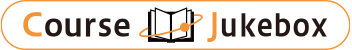### CourseDetailDegree
Bachelor
2
Course delivery methods
face-to-face
Subject
Physical sciences, Mathematical sciences, Engineering & technology
Program
School
School of Science and Engineering
Department
Bachelor's Program in Interdisciplinary Engineering
Campus
Tsukuba Campus
Classroom
3A213
Course Offering Year
2023-2024
Course Offering Month
October - December
Weekday and Period
Tue3,4
Capacity
Credits
2.0
Language
English
Course Number
FJ26104

### Thermodynamics I University of Tsukuba

#### Course Overview

Thermodynamics is one of the essential physics to discuss energy conservation for engineer in various fields. The aim of this lecture is to master the basics of the first and second laws of thermodynamics. The specific goal is to be able to appropriately express the first law of thermodynamics for the system, to be able to discuss changes in entropy based on the second law of thermodynamics, and to combine these basic matters. The heat efficiency of the heat engine can be derived.

#### Learning Achievement

1. Familiarize yourself with mathematical expressions using calculus of basic thermodynamic relations, and understand the difference between minute quantities and finite quantities.
2. Understand the physical meaning of the first law of thermodynamics, and express it mathematically using state quantities such as internal energy and entropy.
3. Explain the irreversible process and the law of increasing entropy based on the understanding of the second law of thermodynamics.
4. Familiarize yourself with the equation transformation starting from the first law of thermodynamics, and can derive the thermal efficiency of the Carnot cycle while making full use of the p-V diagram and the TS diagram.
5. Explain the irreversible process and the law of increasing entropy based on the understanding of the second law of thermodynamics.

#### Competence

Related to 2. Understanding ability of physical phenomena.

#### Course prerequisites

Exercises and homework: 40%
Exams: 60%

#### Course schedule

Week 1: Discovery of thermodynamics-a historical perspective
Week 2: Basic concepts (state, process, properties)
Week 3: The first law of thermodynamics (closed system)
Week 4: The first law of thermodynamics (open system)
Week 5: The second law of thermodynamics
Week 6: The third law of thermodynamics-Entropy (1)
Week 7: The third law of thermodynamics-Entropy (2)
Week 8: How to measure work potential (exergy analysis)
Week 9: Special topic-statistical molecular thermodynamics
Week 10: Review exercise questions and answers

#### Course type

Lectures and Class Exercises

SHEN Biao

#### Other information

Thermodynamics is one of the essential physics to discuss energy conservation for engineer in various fields. The aim of this lecture is to master the basics of the first and second laws of thermodynamics. The specific goal is to be able to appropriately express the first law of thermodynamics for the system, to be able to discuss changes in entropy based on the second law of thermodynamics, and to combine these basic matters. The heat efficiency of the heat engine can be derived.

#### Site for Inquiry

Link to the syllabus provided by the university